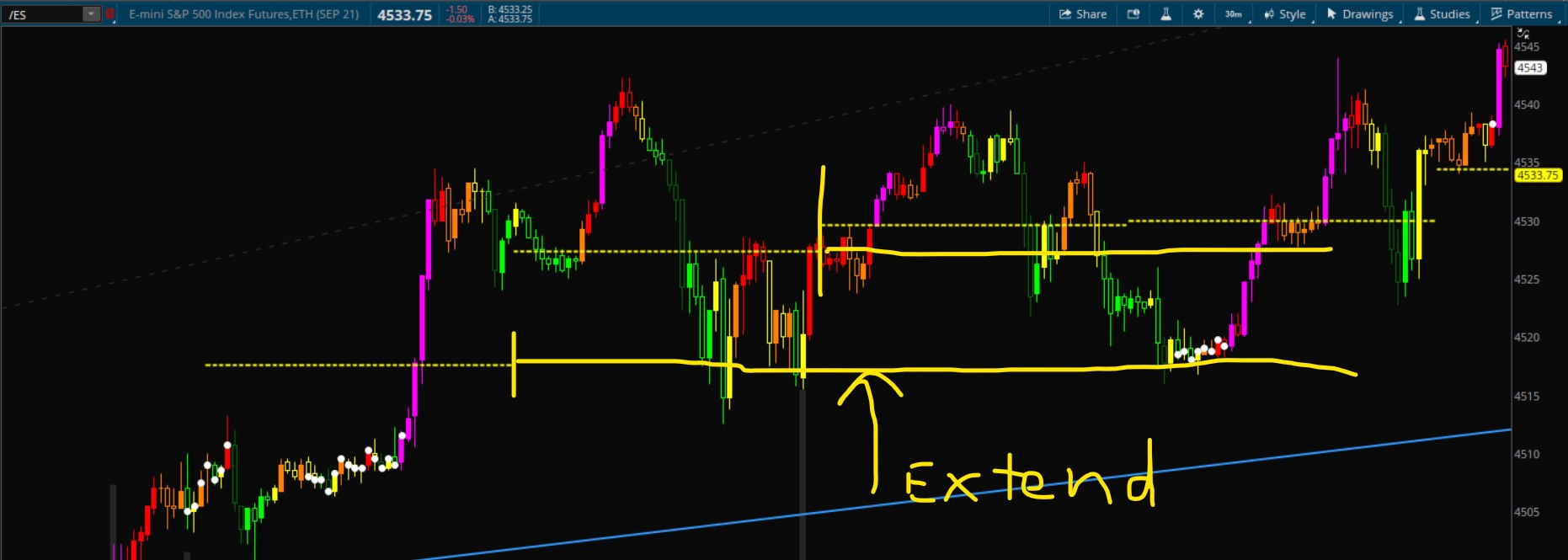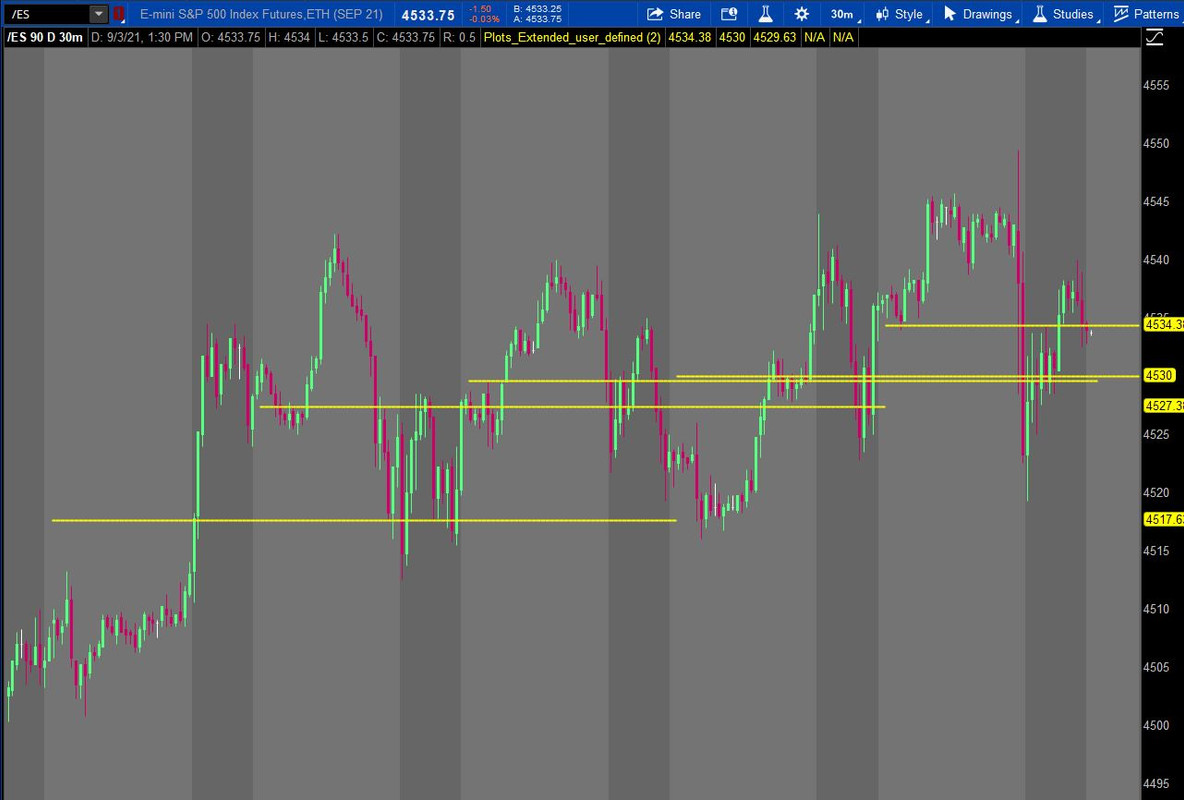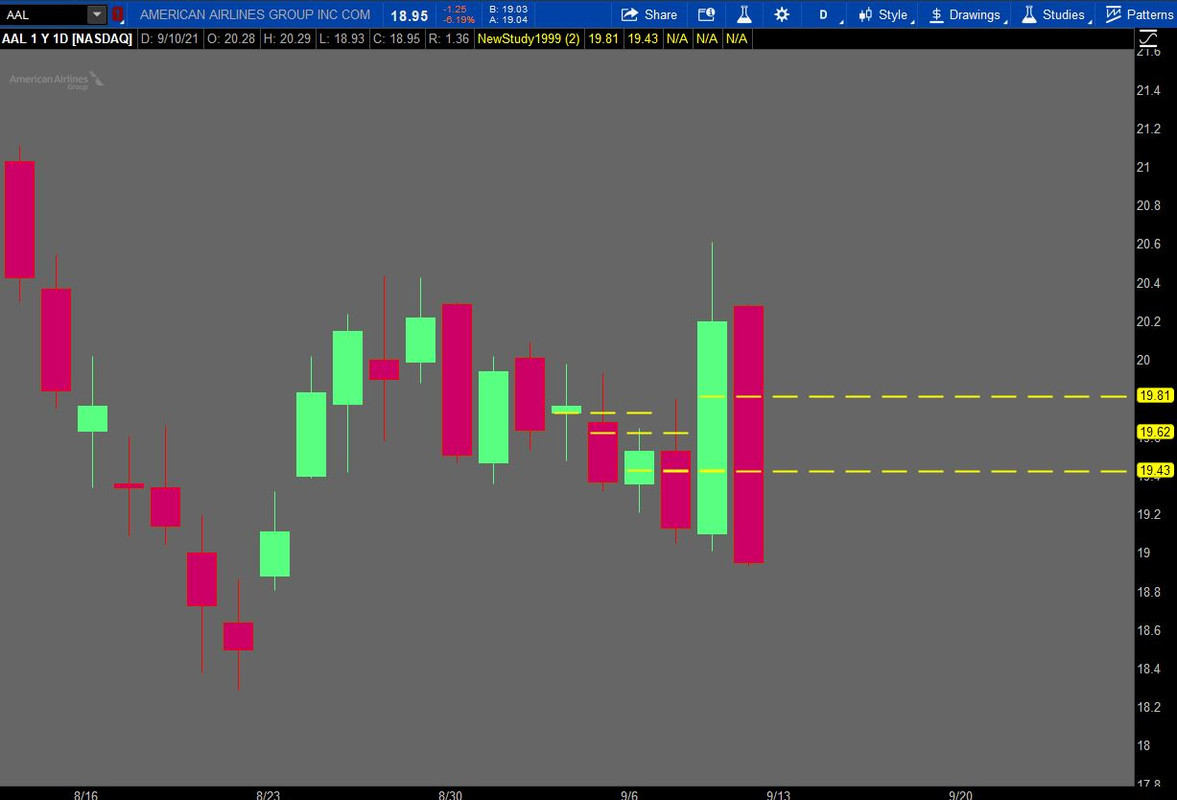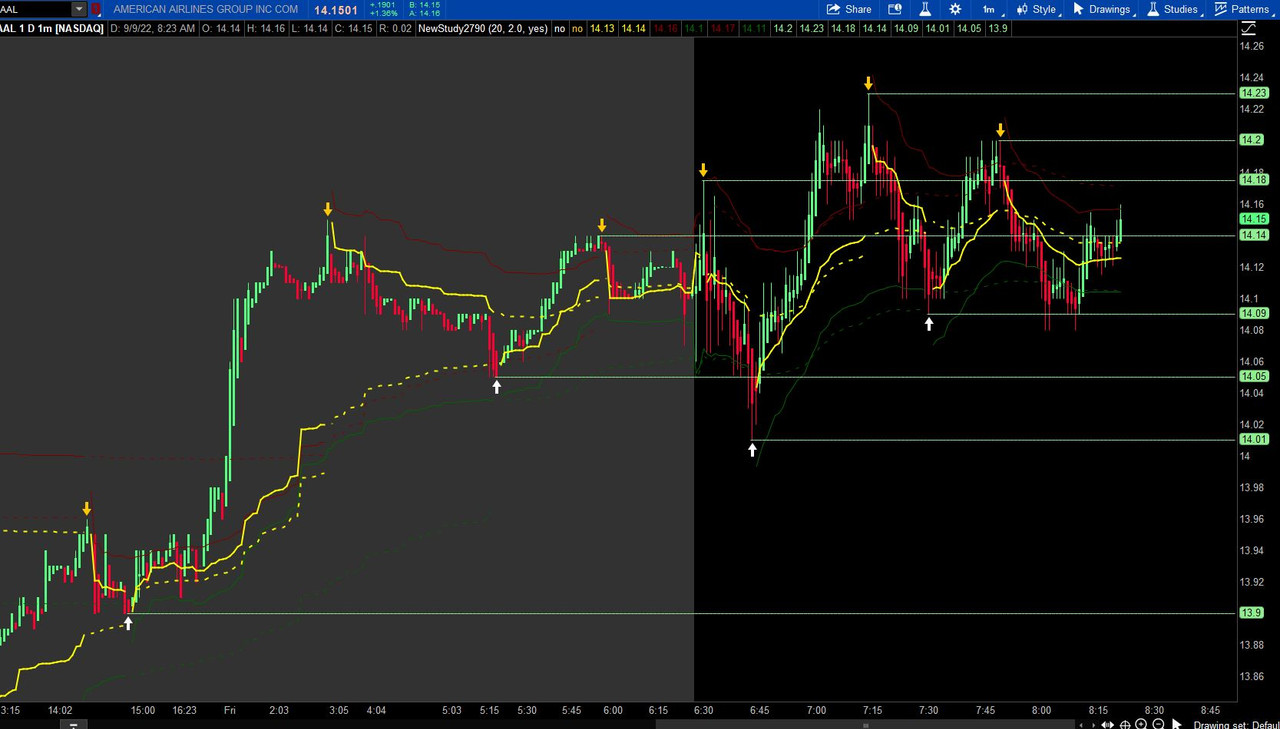# Can Horizontal Daily line be extended for 2 days?

#### chewie76

##### Well-known member
VIP
VIP Enthusiast
I have a code for a daily pivot. The line is one day. Is there any way to take that horizontal line and extend the line to end on the next day or two? Below is the code. Not sure how to get the lines to be two or three days long. If you can help, it would be greatly appreciated!!!Code:
``````declare upper;
input aggregationPeriod = AggregationPeriod.day;
input plot_limit = 10;

def bar = BarNumber();
def high = high(period = aggregationPeriod);
def low = low(period = aggregationPeriod);
def CDP1 = (High + Low) / 2;

def countt = if IsNaN(CDP1) and !IsNaN(CDP1) then 1 else countt + 1;
def cond = CDP1;
rec dataCount = CompoundValue(1, if !IsNaN(cond) then dataCount + 1 else dataCount, 0);

def counth = if IsNaN(CDP1) and !IsNaN(CDP1) then 1 else counth + 1;
plot CDP = if HighestAll(dataCount) - dataCount <= plot_limit - 1 then CDP1 else Double.NaN;

plot CDPEXTEND = if IsNaN(CDP1) then GetValue(CDP1, counth) else Double.NaN;
CDP.SetDefaultColor(Color.YELLOW);
CDP.SetLineWeight(2);
CDP.setpaintingstrategy(paintingstrategy.DASHES);

CDPEXTEND.SetDefaultColor(Color.YELLOW);
CDPEXTEND.SetLineWeight(2);
CDPEXTEND.setpaintingstrategy(paintingstrategy.DASHES);``````

Solution
I have a code for a daily pivot. The line is one day. Is there any way to take that horizontal line and extend the line to end on the next day or two? Below is the code. Not sure how to get the lines to be two or three days long. If you can help, it would be greatly appreciated!!!Code:
``````declare upper;
input aggregationPeriod = AggregationPeriod.day;
input plot_limit = 10;

def bar = BarNumber();
def high = high(period = aggregationPeriod);
def low = low(period = aggregationPeriod);
def CDP1 = (High + Low) / 2;

def countt = if IsNaN(CDP1) and !IsNaN(CDP1) then 1 else countt + 1;
def cond = CDP1;
rec dataCount = CompoundValue(1, if !IsNaN(cond) then dataCount + 1 else dataCount, 0);

def counth = if...``````
I have a code for a daily pivot. The line is one day. Is there any way to take that horizontal line and extend the line to end on the next day or two? Below is the code. Not sure how to get the lines to be two or three days long. If you can help, it would be greatly appreciated!!!Code:
``````declare upper;
input aggregationPeriod = AggregationPeriod.day;
input plot_limit = 10;

def bar = BarNumber();
def high = high(period = aggregationPeriod);
def low = low(period = aggregationPeriod);
def CDP1 = (High + Low) / 2;

def countt = if IsNaN(CDP1) and !IsNaN(CDP1) then 1 else countt + 1;
def cond = CDP1;
rec dataCount = CompoundValue(1, if !IsNaN(cond) then dataCount + 1 else dataCount, 0);

def counth = if IsNaN(CDP1) and !IsNaN(CDP1) then 1 else counth + 1;
plot CDP = if HighestAll(dataCount) - dataCount <= plot_limit - 1 then CDP1 else Double.NaN;

plot CDPEXTEND = if IsNaN(CDP1) then GetValue(CDP1, counth) else Double.NaN;
CDP.SetDefaultColor(Color.YELLOW);
CDP.SetLineWeight(2);
CDP.setpaintingstrategy(paintingstrategy.DASHES);

CDPEXTEND.SetDefaultColor(Color.YELLOW);
CDPEXTEND.SetLineWeight(2);
CDPEXTEND.setpaintingstrategy(paintingstrategy.DASHES);``````

There is not a simple adjustment to your code to extend these lines, as that requires a separate plot statement for each line.

Here is one way to extend these lines as you wish at the input extend, currently shown below at 2. If you want more lines than the five(5) in this script, just copy/paste/edit the plot statements as shown in the following script as many times as needed.Ruby:
``````declare upper;

script x {
input aggregationPeriod = AggregationPeriod.DAY;
def ymd = GetYYYYMMDD();
def candles  = !isnan(close);
def capture  = candles and ymd != ymd;
def dayCount = CompoundValue(1, if capture then dayCount + 1 else dayCount, 0);
def thisDay  = (HighestAll(dayCount) - dayCount) ;
def bar = BarNumber();
def high = high(period = aggregationPeriod);
def low = low(period = aggregationPeriod);
input count = 1;
input extend = 2;
def CDPext = if thisDay == count then (high + low) / 2 else CDPext;

plot CDP = if Between(thisDay, count - extend, count) and CDPext then CDPext else Double.NaN;
}
input extend = 2;
plot cdp1 = x(count = 1, extend = extend);
plot cdp2 = x(count = 2, extend = extend);
plot cdp3 = x(count = 3, extend = extend);
plot cdp4 = x(count = 4, extend = extend);
plot cdp5 = x(count = 5, extend = extend);

cdp1.SetDefaultColor(Color.YELLOW);
cdp1.SetLineWeight(2);
cdp1.setpaintingstrategy(paintingstrategy.DASHES);
CDP2.SetDefaultColor(Color.YELLOW);
CDP2.SetLineWeight(2);
CDP2.setpaintingstrategy(paintingstrategy.DASHES);
CDP3.SetDefaultColor(Color.YELLOW);
CDP3.SetLineWeight(2);
CDP3.setpaintingstrategy(paintingstrategy.DASHES);
CDP4.SetDefaultColor(Color.YELLOW);
CDP4.SetLineWeight(2);
CDP4.setpaintingstrategy(paintingstrategy.DASHES);
CDP5.SetDefaultColor(Color.YELLOW);
CDP5.SetLineWeight(2);
CDP5.setpaintingstrategy(paintingstrategy.DASHES);``````

Last edited:
There is not a simple adjustment to your code to extend these lines, as that requires a separate plot statement for each line.

Here is one way to extend these lines as you wish at the input extend, currently shown below at 2. If you want more lines than the five(5) in this script, just copy/paste/edit the plot statements as shown in the following script as many times as needed.
You are so amazing!!! Any idea why this shows up on 1 hr or less timeframe, I see 2 on the 4 hr and none on the daily chart? Thanks!!!!

Last edited:
You are so amazing!!! Any idea why this shows up on 1 hr or less timeframe, I see 2 on the 4 hr and none on the daily chart? Thanks!!!!

Thank you for the kind comment.

I think this works better now. You should now see daily chart lines. Thanks for letting me know.

The 'thisday snippet' needs to have the barcount of each day to only count when there are bars and thus exclude counting the right expansion. The change to the candles portion of the script to !isnan(close) instead of the previous definition seems to work better on most aggregations.Ruby:
``````declare upper;

script x {
input aggregationPeriod = AggregationPeriod.DAY;
def ymd = GetYYYYMMDD();
def candles  = !isnan(close);#if IsNaN(close) then candles else close;
def capture  = candles and ymd != ymd;
def dayCount = CompoundValue(1, if capture then dayCount + 1 else dayCount, 0);
def thisDay  = (HighestAll(dayCount) - dayCount) ;
def bar = BarNumber();
def high = high(period = aggregationPeriod);
def low = low(period = aggregationPeriod);
input count = 1;
input extend = 2;
def CDPext = if thisDay == count then (high + low) / 2 else CDPext;

plot CDP = if Between(thisDay, count - extend, count) and CDPext then CDPext else Double.NaN;
}
input extend = 2;
plot cdp1 = x(count = 1, extend = extend);
plot cdp2 = x(count = 2, extend = extend);
plot cdp3 = x(count = 3, extend = extend);
plot cdp4 = x(count = 4, extend = extend);
plot cdp5 = x(count = 5, extend = extend);

cdp1.SetDefaultColor(Color.YELLOW);
cdp1.SetLineWeight(2);
cdp1.setpaintingstrategy(paintingstrategy.DASHES);
CDP2.SetDefaultColor(Color.YELLOW);
CDP2.SetLineWeight(2);
CDP2.setpaintingstrategy(paintingstrategy.DASHES);
CDP3.SetDefaultColor(Color.YELLOW);
CDP3.SetLineWeight(2);
CDP3.setpaintingstrategy(paintingstrategy.DASHES);
CDP4.SetDefaultColor(Color.YELLOW);
CDP4.SetLineWeight(2);
CDP4.setpaintingstrategy(paintingstrategy.DASHES);
CDP5.SetDefaultColor(Color.YELLOW);
CDP5.SetLineWeight(2);
CDP5.setpaintingstrategy(paintingstrategy.DASHES);``````

Thank you for the kind comment.

I think this works better now. You should now see daily chart lines. Thanks for letting me know.

The 'thisday snippet' needs to have the barcount of each day to only count when there are bars and thus exclude counting the right expansion. The change to the candles portion of the script to !isnan(close) instead of the previous definition seems to work better on most aggregations.
Looks great!! Thank you so much!!

•SleepyZ
SleepyZ: I would like to incorporate extended horizontal lines for the pivots identified in the Anchored VWAP study below using your approach above, but am failing miserably to duplicate your logic. I did not insert a screenshot but currently there are up/down arrows tied to the pivot points and would like horizontal lines on the last 5 pivots (or so). Don't know if the fold function is blowing up the script function you used above but would appreciate any insight you can offer.

Ruby:
``````#START STUDY#Anchored_VWAP2
#linus, 2014-03-10, v0.1

#hint: VWAP stops anchored off  fractalTrader pivots.

#hint n: Lookback period for finding swing highs, lows.
input n = 20;

#hint ticks: Offset High/Low VWAP lines by this number of ticks.
input ticks = 2.0;

def bnOK = barNumber() > n;

def isHigher = fold i = 1 to n + 1 with p = 1
while p do high > GetValue(high, -i);

def HH = if bnOK and isHigher
and high == Highest(high, n)
then high else Double.NaN;

def isLower = fold j = 1 to n + 1 with q = 1
while q do low < GetValue(low, -j);

def LL = if bnOK and isLower
and low == Lowest(low, n)
then low else Double.NaN;

def PivH = if HH > 0 then HH else Double.NaN;
def PivL = if LL > 0 then LL else Double.NaN;

plot Up = !isNaN(PivL);
Up.SetPaintingStrategy(PaintingStrategy.BOOLEAN_ARROW_UP);
Up.SetLineWeight(3);
Up.SetDefaultColor(Color.WHITE);

plot Dn = !isNaN(PivH);
Dn.SetPaintingStrategy(PaintingStrategy.BOOLEAN_ARROW_DOWN);
Dn.SetLineWeight(3);
Dn.SetDefaultColor(Color.ORANGE);

def LocH = (high + (tickSize() * ticks)) * volume;
def LocL = (low - (tickSize() * ticks)) * volume;
def LocC = close * volume;

rec PC;
rec VC;
rec PC2;
rec VC2;
rec PH;
rec VH;
rec PL;
rec VL;
rec PH2;
rec VH2;
rec PL2;
rec VL2;

if Dn or Up {
PC = LocC;
VC = volume;
PC2 = PC;
VC2 = VC;
} else {
PC = compoundValue(1, LocC + PC, Double.NaN);
VC = compoundValue(1, volume + VC, Double.NaN);
PC2 = compoundValue(1, LocC + PC2, Double.NaN);
VC2 = compoundValue(1, volume + VC2, Double.NaN);
}

if Dn {
PH = LocH;
VH = volume;
PH2 = PH;
VH2 = VH;
} else {
PH = compoundValue(1, LocH + PH, Double.NaN);
VH = compoundValue(1, volume + VH, Double.NaN);
PH2 = compoundValue(1, LocH + PH2, Double.NaN);
VH2 = compoundValue(1, volume + VH2, Double.NaN);
}
if Up  {
PL = LocL;
VL = volume;
PL2 = PL;
VL2 = VL;
} else {
PL = compoundValue(1, LocL + PL, Double.NaN);
VL = compoundValue(1, volume + VL, Double.NaN);
PL2 = compoundValue(1, LocL + PL2, Double.NaN);
VL2 = compoundValue(1, volume + VL2, Double.NaN);
}

plot VwapC = if Dn or Up then Double.NaN else PC / VC;
plot VwapC2 = if Dn or Up then Double.NaN else PC2 / VC2;
plot VwapH = if Dn then Double.NaN else PH / VH;
plot VwapL = if Up then Double.NaN else PL / VL;
plot VwapH2 = if Dn then Double.NaN else PH2 / VH2;
plot VwapL2 = if Up then Double.NaN else PL2 / VL2;

# directional versus rotational logic tests
def C_1 = if (VwapC > VwapC,1,-1);
def C_2 = if (VwapC2 > VwapC2,1,-1);
def C_3 = if (VwapC > VwapC2,1,-1);
def H_1 = if (VwapH > VwapH,1,-1);
def H_2 = if (VwapH2 > VwapH2,1,-1);
def H_3 = if (VwapH > VwapH2,1,-1);
def L_1 = if (VwapL > VwapL,1,-1);
def L_2 = if (VwapL2 > VwapL2,1,-1);
def L_3 = if (VwapL > VwapL2,1,-1);

def context = C_1+C_2+C_3+H_1+H_2+H_3+L_1+L_2+L_3;

AddLabel(yes,if context < -8 then "Directional Down" else if context > 8 then "Directional Up" else "Rotational", (if context < -8 then Color.RED else if context > 8 then Color.GREEN else Color.YELLOW));

VwapC.SetDefaultColor(Color.YELLOW);
VwapC.SetLineWeight(2);
VwapC.HideBubble();

VwapC2.SetDefaultColor(Color.YELLOW);
VwapC2.SetLineWeight(2);
VwapC2.SetStyle(Curve.SHORT_DASH);
VwapC2.HideBubble();

VwapH.SetDefaultColor(Color.DARK_RED);
VwapH.HideBubble();

VwapL.SetDefaultColor(Color.DARK_GREEN);
VwapL.HideBubble();

VwapH2.SetDefaultColor(Color.DARK_RED);
VwapH2.SetStyle(Curve.SHORT_DASH);
VwapH2.HideBubble();

VwapL2.SetDefaultColor(Color.DARK_GREEN);
VwapL2.SetStyle(Curve.SHORT_DASH);
VwapL2.HideBubble();

#END STUDY``````

SleepyZ: I would like to incorporate extended horizontal lines for the pivots identified in the Anchored VWAP study below using your approach above, but am failing miserably to duplicate your logic. I did not insert a screenshot but currently there are up/down arrows tied to the pivot points and would like horizontal lines on the last 5 pivots (or so). Don't know if the fold function is blowing up the script function you used above but would appreciate any insight you can offer.

Ruby:
``````#START STUDY#Anchored_VWAP2
#linus, 2014-03-10, v0.1

#hint: VWAP stops anchored off  fractalTrader pivots.

#hint n: Lookback period for finding swing highs, lows.
input n = 20;

#hint ticks: Offset High/Low VWAP lines by this number of ticks.
input ticks = 2.0;

def bnOK = barNumber() > n;

def isHigher = fold i = 1 to n + 1 with p = 1
while p do high > GetValue(high, -i);

def HH = if bnOK and isHigher
and high == Highest(high, n)
then high else Double.NaN;

def isLower = fold j = 1 to n + 1 with q = 1
while q do low < GetValue(low, -j);

def LL = if bnOK and isLower
and low == Lowest(low, n)
then low else Double.NaN;

def PivH = if HH > 0 then HH else Double.NaN;
def PivL = if LL > 0 then LL else Double.NaN;

plot Up = !isNaN(PivL);
Up.SetPaintingStrategy(PaintingStrategy.BOOLEAN_ARROW_UP);
Up.SetLineWeight(3);
Up.SetDefaultColor(Color.WHITE);

plot Dn = !isNaN(PivH);
Dn.SetPaintingStrategy(PaintingStrategy.BOOLEAN_ARROW_DOWN);
Dn.SetLineWeight(3);
Dn.SetDefaultColor(Color.ORANGE);

def LocH = (high + (tickSize() * ticks)) * volume;
def LocL = (low - (tickSize() * ticks)) * volume;
def LocC = close * volume;

rec PC;
rec VC;
rec PC2;
rec VC2;
rec PH;
rec VH;
rec PL;
rec VL;
rec PH2;
rec VH2;
rec PL2;
rec VL2;

if Dn or Up {
PC = LocC;
VC = volume;
PC2 = PC;
VC2 = VC;
} else {
PC = compoundValue(1, LocC + PC, Double.NaN);
VC = compoundValue(1, volume + VC, Double.NaN);
PC2 = compoundValue(1, LocC + PC2, Double.NaN);
VC2 = compoundValue(1, volume + VC2, Double.NaN);
}

if Dn {
PH = LocH;
VH = volume;
PH2 = PH;
VH2 = VH;
} else {
PH = compoundValue(1, LocH + PH, Double.NaN);
VH = compoundValue(1, volume + VH, Double.NaN);
PH2 = compoundValue(1, LocH + PH2, Double.NaN);
VH2 = compoundValue(1, volume + VH2, Double.NaN);
}
if Up  {
PL = LocL;
VL = volume;
PL2 = PL;
VL2 = VL;
} else {
PL = compoundValue(1, LocL + PL, Double.NaN);
VL = compoundValue(1, volume + VL, Double.NaN);
PL2 = compoundValue(1, LocL + PL2, Double.NaN);
VL2 = compoundValue(1, volume + VL2, Double.NaN);
}

plot VwapC = if Dn or Up then Double.NaN else PC / VC;
plot VwapC2 = if Dn or Up then Double.NaN else PC2 / VC2;
plot VwapH = if Dn then Double.NaN else PH / VH;
plot VwapL = if Up then Double.NaN else PL / VL;
plot VwapH2 = if Dn then Double.NaN else PH2 / VH2;
plot VwapL2 = if Up then Double.NaN else PL2 / VL2;

# directional versus rotational logic tests
def C_1 = if (VwapC > VwapC,1,-1);
def C_2 = if (VwapC2 > VwapC2,1,-1);
def C_3 = if (VwapC > VwapC2,1,-1);
def H_1 = if (VwapH > VwapH,1,-1);
def H_2 = if (VwapH2 > VwapH2,1,-1);
def H_3 = if (VwapH > VwapH2,1,-1);
def L_1 = if (VwapL > VwapL,1,-1);
def L_2 = if (VwapL2 > VwapL2,1,-1);
def L_3 = if (VwapL > VwapL2,1,-1);

def context = C_1+C_2+C_3+H_1+H_2+H_3+L_1+L_2+L_3;

AddLabel(yes,if context < -8 then "Directional Down" else if context > 8 then "Directional Up" else "Rotational", (if context < -8 then Color.RED else if context > 8 then Color.GREEN else Color.YELLOW));

VwapC.SetDefaultColor(Color.YELLOW);
VwapC.SetLineWeight(2);
VwapC.HideBubble();

VwapC2.SetDefaultColor(Color.YELLOW);
VwapC2.SetLineWeight(2);
VwapC2.SetStyle(Curve.SHORT_DASH);
VwapC2.HideBubble();

VwapH.SetDefaultColor(Color.DARK_RED);
VwapH.HideBubble();

VwapL.SetDefaultColor(Color.DARK_GREEN);
VwapL.HideBubble();

VwapH2.SetDefaultColor(Color.DARK_RED);
VwapH2.SetStyle(Curve.SHORT_DASH);
VwapH2.HideBubble();

VwapL2.SetDefaultColor(Color.DARK_GREEN);
VwapL2.SetStyle(Curve.SHORT_DASH);
VwapL2.HideBubble();

#END STUDY``````

That version of Linus study did not update pivots real time but one would have to refresh the screen to get the pivot plot.

So, I used the version I fixed (and was in that lengthy thread) in the following script that has the horizontal lines at the pivots that you can choose to extend to the right edge. You can make more of these lines by using the copying and pasting the last of ph3/pl3 and change the numbers incrementally.Ruby:
``````#START STUDY
#Anchored_VWAPv3
#linus, 2014-03-10, v0.1

#10:24 linus: it carries over the previous pivot's lines for high, low and close. (it plots vwaps of the high, low and close that are reset each time a new pivot is found.)
#10:25 linus: i wrote it to experiment with vwap as stops. (the high and low vwaps that can be offset by the ticks input.)
#10:25 linus: but it should serve as an example of how to reset the vwaps based on a signal.
#10:35 linus: #hint: VWAP stops anchored off  fractalTrader pivots.
#10:37 linus: the code calculates the pivots as PivH and PivL, and then restarts the high, low and close vwaps when it finds a new pivot.  Otherwise it continues to calculate the high, low and close vwaps.
#10:37 linus: the dashed vwap plots are the saved from the previous pivot, and the solid vwap plots are since the last pivot.
#20220708 used missing logic from Mobius fractal pivots
#20220909 modified to plot horizontal lines at pivot that can be extended through a script function.

#hint: VWAP stops anchored off  fractalTrader pivots.

#hint n: Lookback period for finding swing highs, lows.

input n = 20;

#hint ticks: Offset High/Low VWAP lines by this number of ticks.
input ticks  = 2.0;
def bn       = barnumber();
def na       = double.nan;
def bnOK     = Bn > n;

def isHigher = fold i = 1 to n + 1 with p = 1
while p do high > GetValue(high, -i);

def HH       = if bnOK and isHigher and
high == Highest(high, n)
then high else na;

def isLower  = fold j = 1 to n + 1 with q = 1
while q do low < GetValue(low, -j);

def LL       = if bnOK and isLower and
low == Lowest(low, n)
then low else na;

def PHBar    = if !IsNaN(HH)
then bn
else PHBar;

def PLBar    = if !IsNaN(LL)
then bn
else PLBar;

def PHL     = if !IsNaN(HH)
then HH
else PHL;

def PLL     = if !IsNaN(LL)
then LL
else PLL;

def priorPHBar = if PHL != PHL
then PHBar
else priorPHBar;

def priorPLBar = if PLL != PLL
then PLBar
else priorPLBar;

def HighPivots = Bn >= HighestAll(priorPHBar);
def LowPivots  = Bn >= HighestAll(priorPLBar);

def PivH       = if !IsNaN(HH) > 0 then HighPivots else na;
def PivL       = if !IsNaN(LL) > 0 then LowPivots  else na;

plot Up        = Bn == PLBar;
Up.SetPaintingStrategy(PaintingStrategy.BOOLEAN_ARROW_UP);
Up.SetLineWeight(3);
Up.SetDefaultColor(Color.WHITE);

plot Dn        = Bn == PHBar;
Dn.SetPaintingStrategy(PaintingStrategy.BOOLEAN_ARROW_DOWN);
Dn.SetLineWeight(3);
Dn.SetDefaultColor(Color.ORANGE);

def LocH = (high + (TickSize() * ticks)) * volume;
def LocL = (low  - (TickSize() * ticks)) * volume;
def LocC = close * volume;

rec PC;
rec VC;
rec PC2;
rec VC2;
rec PH;
rec VH;
rec PL;
rec VL;
rec PH2;
rec VH2;
rec PL2;
rec VL2;

if Dn or Up {
PC = LocC;
VC = volume;
PC2 = PC;
VC2 = VC;
} else {
PC = CompoundValue(1, LocC + PC, na);
VC = CompoundValue(1, volume + VC, na);
PC2 = CompoundValue(1, LocC + PC2, na);
VC2 = CompoundValue(1, volume + VC2, na);
}

if Dn {
PH = LocH;
VH = volume;
PH2 = PH;
VH2 = VH;
} else {
PH = CompoundValue(1, LocH + PH, na);
VH = CompoundValue(1, volume + VH, na);
PH2 = CompoundValue(1, LocH + PH2, na);
VH2 = CompoundValue(1, volume + VH2, na);
}
if Up  {
PL = LocL;
VL = volume;
PL2 = PL;
VL2 = VL;
} else {
PL = CompoundValue(1, LocL + PL, na);
VL = CompoundValue(1, volume + VL, na);
PL2 = CompoundValue(1, LocL + PL2, na);
VL2 = CompoundValue(1, volume + VL2, na);
}

plot VwapC = if Dn or Up then na else PC / VC;
plot VwapC2 = if Dn or Up then na else PC2 / VC2;
plot VwapH = if Dn then na else PH / VH;
plot VwapL = if Up then na else PL / VL;
plot VwapH2 = if Dn then na else PH2 / VH2;
plot VwapL2 = if Up then na else PL2 / VL2;

VwapC.SetDefaultColor(Color.YELLOW);
VwapC.SetLineWeight(2);
VwapC.HideBubble();

VwapC2.SetDefaultColor(Color.YELLOW);
VwapC2.SetLineWeight(2);
VwapC2.SetStyle(Curve.SHORT_DASH);
VwapC2.HideBubble();

VwapH.SetDefaultColor(Color.DARK_RED);
VwapH.HideBubble();

VwapL.SetDefaultColor(Color.DARK_GREEN);
VwapL.HideBubble();

VwapH2.SetDefaultColor(Color.DARK_RED);
VwapH2.SetStyle(Curve.SHORT_DASH);
VwapH2.HideBubble();

VwapL2.SetDefaultColor(Color.DARK_GREEN);
VwapL2.SetStyle(Curve.SHORT_DASH);
VwapL2.HideBubble();
#END Linus STUDY

#============================================================================
#Addition of Limited Horizontal Lines at the pivot points. These lines can be extended to the right edge at input extend.

script a {

def n = 20;

#hint ticks: Offset High/Low VWAP lines by this number of ticks.
def ticks  = 2.0;
def bn       = barnumber();
def na       = double.nan;
def bnOK     = Bn > n;

def isHigher = fold i = 1 to n + 1 with p = 1
while p do high > GetValue(high, -i);

def HH       = if bnOK and isHigher and
high == Highest(high, n)
then high else na;

def isLower  = fold j = 1 to n + 1 with q = 1
while q do low < GetValue(low, -j);

def LL       = if bnOK and isLower and
low == Lowest(low, n)
then low else na;

def PHBar    = if !IsNaN(HH)
then bn
else PHBar;

def PLBar    = if !IsNaN(LL)
then bn
else PLBar;

def PHL     = if !IsNaN(HH)
then HH
else PHL;

def PLL     = if !IsNaN(LL)
then LL
else PLL;

def priorPHBar = if PHL != PHL
then PHBar
else priorPHBar;

def priorPLBar = if PLL != PLL
then PLBar
else priorPLBar;

def HighPivots = Bn >= HighestAll(priorPHBar);
def LowPivots  = Bn >= HighestAll(priorPLBar);

def PivH       = if !IsNaN(HH) > 0 then HighPivots else na;
def PivL       = if !IsNaN(LL) > 0 then LowPivots  else na;

input pivotplot = 0;
input extend    = yes;

#PH ============================================================================
def phcond   = if isnan(close) then phcond else if Bn == PHBar then high else phcond;
def candles  = !IsNaN(close);
def capture  = phcond != phcond;
def dayCount = CompoundValue(1, if capture then dayCount + 1 else dayCount, 0);
def thisDay  = (HighestAll(dayCount)- dayCount);

def phext = if isnan(close) then phext else if thisday==pivotplot then phcond else phext;

plot pivhi = if thisday < pivotplot + 1 then if extend then phext else phcond else double.nan;
pivhi.setpaintingStrategy(paintingStrategy.HORIZONTAL);
pivhi.SetDefaultColor(Color.Light_green);

#PL =============================================================================
def plcond   = if isnan(close) then plcond else if Bn == PLBar then low else plcond;
def candles1 = !IsNaN(close);
def capture1 = plcond != plcond;
def dayCount1= CompoundValue(1, if capture1 then dayCount1 + 1 else dayCount1, 0);
def thisDay1  = (HighestAll(dayCount1)- dayCount1) ;

def plext = if isnan(close) then plext else if thisday1==pivotplot then plcond else plext;

plot pivlo = if thisday1 < pivotplot + 1 then if extend then plext else plcond else double.nan;
pivlo.setpaintingStrategy(paintingStrategy.HORIZONTAL);
pivlo.SetDefaultColor(Color.light_red);

}

#Horizontal Lines at Pivot Points ==============================================
input extend = yes;

#Pivot Highs ====================================================================
plot ph0 = a(0,  extend = extend);
ph0.setpaintingStrategy(paintingStrategy.HORIZONTAL);
ph0.SetDefaultColor(Color.Light_green);
plot ph1 = a(1, extend = extend);
ph1.setpaintingStrategy(paintingStrategy.HORIZONTAL);
ph1.SetDefaultColor(Color.Light_green);
plot ph2_ = a(2, extend = extend);
ph2_.setpaintingStrategy(paintingStrategy.HORIZONTAL);
ph2_.SetDefaultColor(Color.Light_green);
plot ph3 = a(3, extend = extend);
ph3.setpaintingStrategy(paintingStrategy.HORIZONTAL);
ph3.SetDefaultColor(Color.Light_green);

#Pivot Lows =====================================================================
plot pl0 = a(0, extend).pivlo;
pl0.setpaintingStrategy(paintingStrategy.HORIZONTAL);
pl0.SetDefaultColor(Color.Light_green);
plot pl1 = a(1, extend).pivlo;
pl1.setpaintingStrategy(paintingStrategy.HORIZONTAL);
pl1.SetDefaultColor(Color.Light_green);
plot pl2_ = a(2, extend).pivlo;
pl2_.setpaintingStrategy(paintingStrategy.HORIZONTAL);
pl2_.SetDefaultColor(Color.Light_green);
plot pl3 = a(3, extend).pivlo;
pl3.setpaintingStrategy(paintingStrategy.HORIZONTAL);
pl3.SetDefaultColor(Color.Light_green);``````

Last edited:
Works perfectly and I see where I screwed up. Kind thanks for the masterful coding

•SleepyZ

### Not the exact question you're looking for?

87k+ Posts
236 Online## The Market Trading Game Changer

Join 2,500+ subscribers inside the useThinkScript VIP Membership Club
• Exclusive indicators
• Proven strategies & setups
• Private Discord community
• Exclusive members-only content
• 1 full year of unlimited support

What is useThinkScript?

useThinkScript is the #1 community of stock market investors using indicators and other tools to power their trading strategies. Traders of all skill levels use our forums to learn about scripting and indicators, help each other, and discover new ways to gain an edge in the markets.

How do I get started?

We get it. Our forum can be intimidating, if not overwhelming. With thousands of topics, tens of thousands of posts, our community has created an incredibly deep knowledge base for stock traders. No one can ever exhaust every resource provided on our site.

If you are new, or just looking for guidance, here are some helpful links to get you started.

What are the benefits of VIP Membership?